## A proton is released from rest at the origin in a uniform electric field that is directed in the positive xx direction with magnitude 950 \t

Question

A proton is released from rest at the origin in a uniform electric field that is directed in the positive xx direction with magnitude 950 \text{ N/C}950 N/C. What is the change in the electric potential energy of the proton-field system when the proton travels to x

in progress 0
3 months 2021-08-18T07:15:07+00:00 1 Answers 28 views 0

The change in potential energy is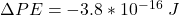Explanation:

From the question we are told that

The  magnitude of the uniform electric field  is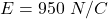The  distance traveled by the electron is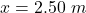Generally the force on this electron is  mathematically represented as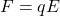Where F is the force and  q is the charge on the electron which is  a constant value of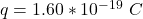Thus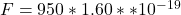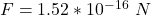Generally the work energy theorem can be mathematically represented as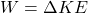Where W is the workdone on the electron by the  Electric field and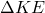is the change in kinetic energy

Also  workdone on the electron can also  be represented as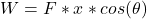Where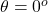considering that the movement of the electron is along the x-axis

So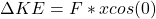substituting values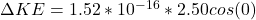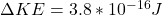Now From the law of energy conservation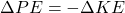Where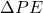is the change  in  potential energy

Thus